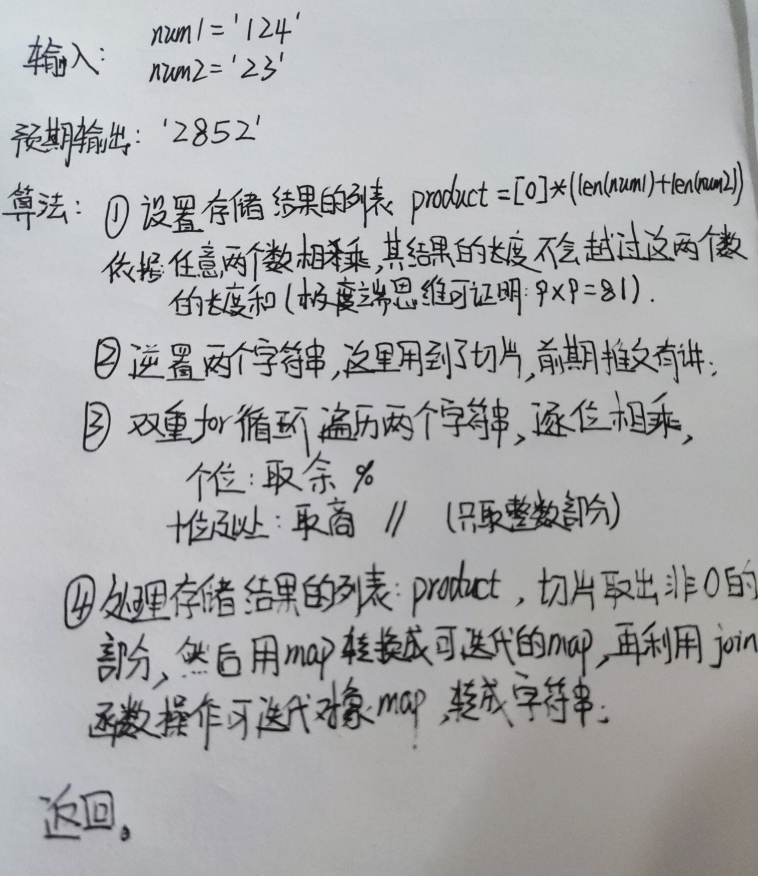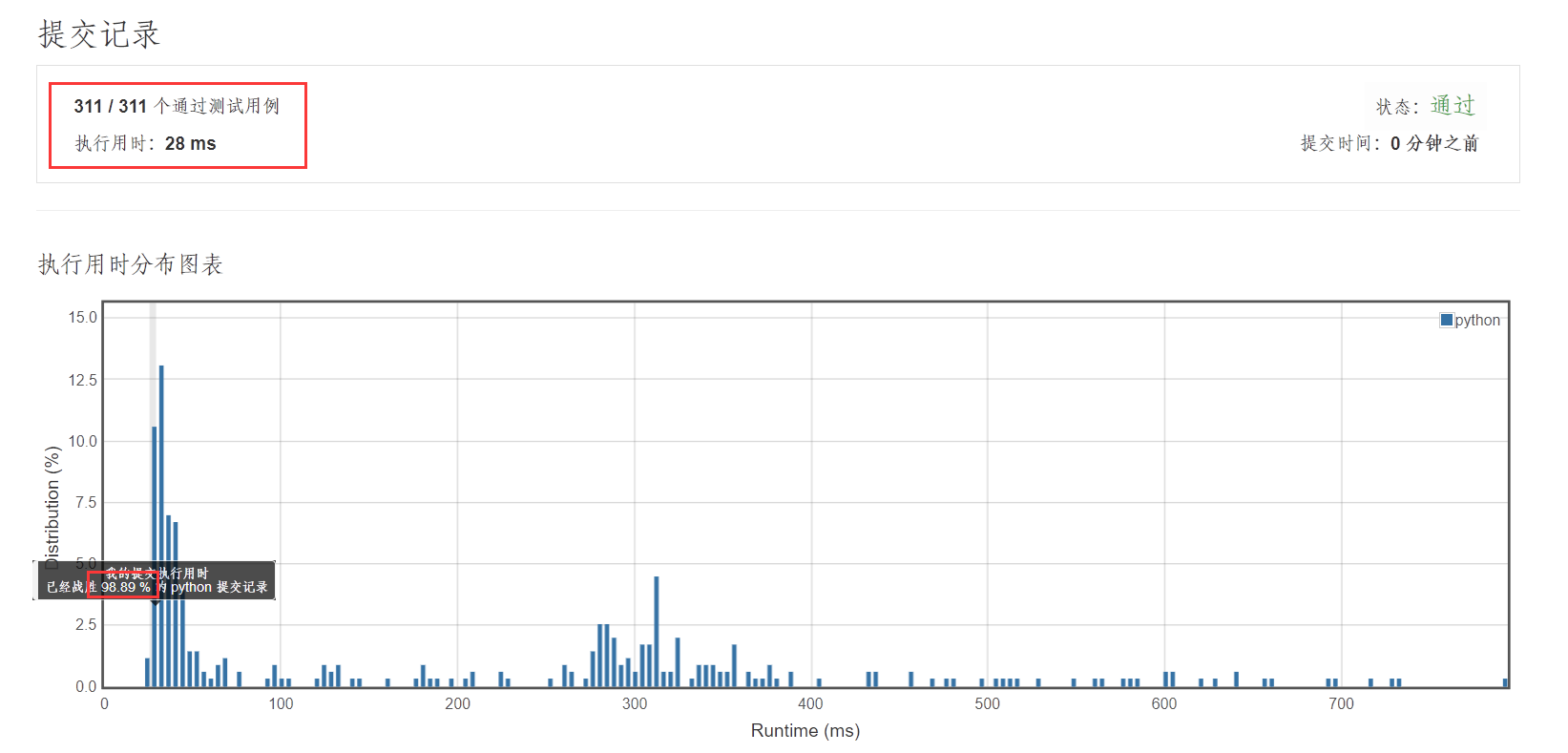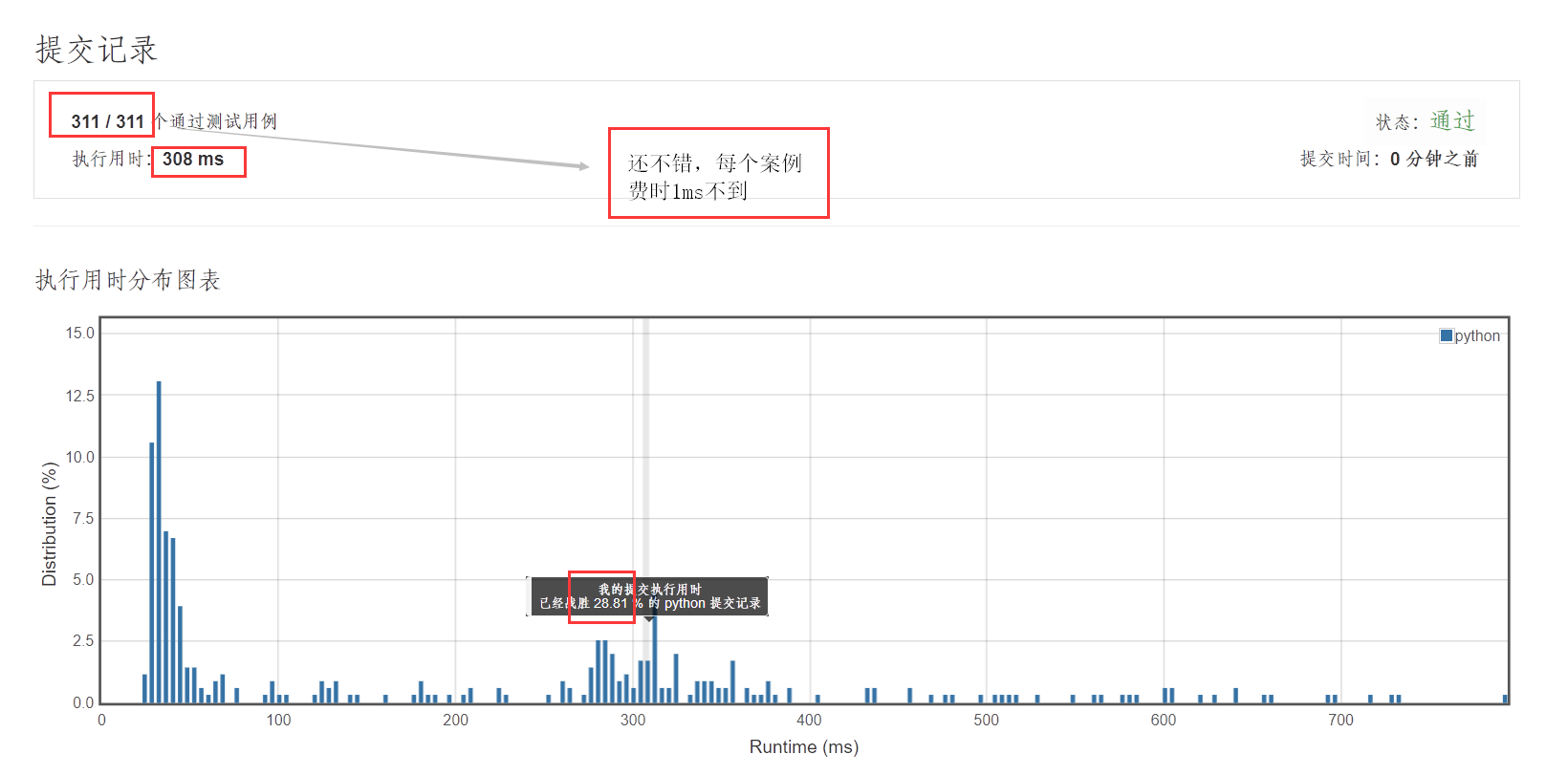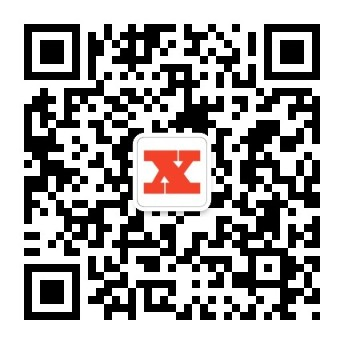LeetCode011:字符串相乘

一、写在前面

LeetCode 第二题两数之和传输门：LeetCode010 : 盛最多水的容器

二、今日题目

(1) num1 和 num2 的长度小于110。
(2) num1 和 num2 只包含数字 0-9。
(3)num1 和 num2 均不以零开头，除非是数字 0 本身。
(4) 不能使用任何标准库的大数类型（比如 BigInteger）或直接将输入转换为整数来处理。

输入: num1 = "2", num2 = "3"



三、 分析四、解题

• 捷径的方法：
调包调包调包
class Solution(object):
def multiply(self, num1, num2):
"""
:type num1: str
:type num2: str
:rtype: str
"""
# str->int
num1 = int(num1)
num2 = int(num2)
# 求积
result = num1*num2
# int->str,返回
return str(result)

• 提交结果• 正确思想
时间复杂度：O(n^2)
class Solution(object):
def multiply(self, num1, num2):
"""
:type num1: str
:type num2: str
:rtype: str
"""
# 设置存储列表(原理见思路分析)
product =  * (len(num1) + len(num2))
# 下标
pos = len(product) - 1
# 字符串逆转，方便从个位开始遍历
num1 = num1[::-1]
num2 = num2[::-1]
# 遍历被乘数字符串
for n1 in num1:
# 数据存储位置(数量级，第一次个位相乘，最后一位，第二次十位相乘，倒数第二位...)
tempPos = pos
# 遍历乘数字符串
for n2 in num2:
# 单个数据相乘
product[tempPos] += int(n1) * int(n2)
# 取十位及以上量级
product[tempPos - 1] += product[tempPos] // 10
# 取个位
product[tempPos] %= 10
# 前移
tempPos -= 1
# 前移，增加数量级
pos -= 1
# 找到结果列表非零数
pt = 0
while pt < len(product) - 1 and product[pt] == 0:
pt += 1
# 切片取出数据
res_list = product[pt:]
# map转换成迭代对象，用jion函数连接返回
return ''.join(map(str,res_list))

• 提交结果1.考察对字符串的操作处理；
2.考察对数据运行的操作处理。

六、结语